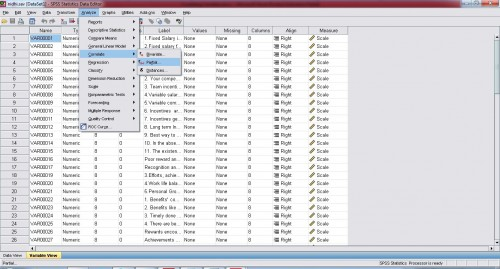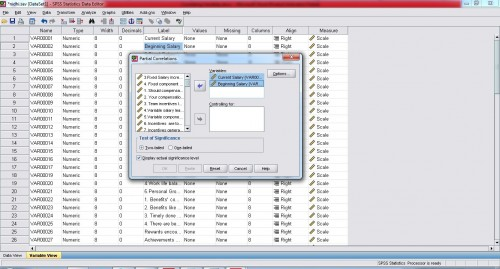# Correlation of variables in SPSS

It measures the correlations between two or more numeric variables. There are two types of correlations; bivariate and partial correlations. While Bivariate Correlations are computed using Pearson/Spearman Correlation Coefficient wherein it gives the measure of correlations between variables or rank orders. The value of correlation coefficient lies between -1 to +1 and value “0” indicates that there is no correlation. On the other hand Partial correlation procedure is applied to calculate partial correlation coefficient in order to describe the relationship between two variables along with adjustments made regarding the effect of one variable on another.

While Pearson Correlation Coefficient is used to calculate linear relationships, i.e. if one variable increases or decreases what is the extent to which other variable also increases or decreases. For example, to determine the relationship between rise in temperature and decrease in level of snow we would use Pearson correlation. On the other hand Spearman Correlation coefficient is used to determine monotonic relationship between variables. For example; to determine the time taken by employees to complete the test depending on the time they have been employed within the firm. However, between the two methods, pearson correlation is found to be more precise method to determine correlations.

## Examples of Correlation

Bivariate: Pearson Correlation coefficient is calculated to determine the relationship (weak/strong) between current salary and beginning salary of employees within the organization.

Partial Correlation: Partial correlation can be applied to study the correlation between current salary and beginning salary along with control variables like; months since hired, previous experience of the employee.

### Bivariate correlations

Please Note:  With respect to One-Tailed and Two tailed test of significance, while in case of one-tailed test, you as a researcher are proposing that there is one possible relationship between the two variables which are being tested and that either the variable is higher than mean or lower than mean, however in case of two-tailed test, we are speculating that these variables can be both higher than average mean or lower than average mean. We generally use Two-Tailed Test as it has better precision.

Click OK to run the procedure.

 Correlations Current Salary Beginning Salary Current Salary Pearson Correlation 1 .573* Sig. (2-tailed) .045 N 255 255 Beginning Salary Pearson Correlation .573* 1 Sig. (2-tailed) .045 N 255 255

**. Correlation is significant at the 0.01 level (2-tailed). (This means the value will be considered significant if is between 0.001 to 0,010, See 2nd example below).

*. Correlation is significant at the 0.05 level (2-tailed). (This means the value will be considered significant if is between 0.010 to 0,050).

The output table shown above provides Pearson Correlations between the pair i.e. Current Salary and Beginning Salary. The results indicate that Beginning salary influences the current salary of the employee (α= .573, p=0.45) (Please Note: The value of Pearson correlation and Sig are complementary to each other, further and therefore both the values are considered important when writing results) where value of Significance (2-Tailed) is less than 0.05 significant at 95% confidence interval. The correlation value (α) and Sig value (p) will always complement each other, i.e. if one value is “acceptable” then the other will be “acceptable” too.

### Partial Correlations using SPSScorrelate and then Partial, which opens a dialog box which is shown” width=”500″ height=”269″> Select Analyze, -> correlate and then Partial, which opens a dialog box which as shown.Select Current Salary and Beginning Salary and move them to VARIABLE list, as shown.Next, Select, Months since Hire and Previous Job Experience and Move them to CONTROLLING FOR list. Click OK to Run the Procedure.
 Correlations Control Variables Current Salary Beginning Salary Months Since Hired & Previous Job Experience Current Salary Correlation 1.000 .711** Significance (2-tailed) .001 df 0 251 Beginning Salary Correlation .711** 1.000 Significance (2-tailed) .001 df 251 0

**. Correlation is significant at the 0.01 level (2-tailed).

*. Correlation is significant at the 0.05 level (2-tailed).

Legends:

• Pearson Correlation: Gives the value for Correlation at confidence interval of 95%
• Sig (2-tailed): Gives the value of significance of correlation between the two variables at 95% confidence interval
• Df: Displays the degree of freedom, i.e. the sample size of the study.

The Output given above (Table 1) shows the table of Partial Correlation Coefficient, the Degree of Freedom, and the significance level for the two correlating variables i.e. current Salary and Beginning salary. The results of partial correlation indicate that control variables Months since hired and Previous job experience influence the current and the beginning salary of the employees. Here α=.711 and p= 0.001 (p < 0.01) which reflects significance at 98% confidence interval.

### Priya Chetty

Partner at Project Guru
Priya is a master in business administration with majors in marketing and finance. She is fluent with data modelling, time series analysis, various regression models, forecasting and interpretation of the data. She has assisted data scientists, corporates, scholars in the field of finance, banking, economics and marketing.